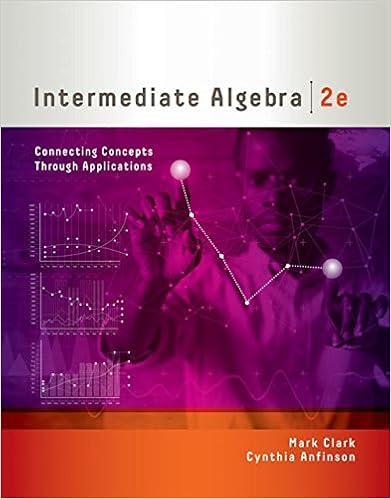# Velocity of ball b after the collision is ms round to

• Homework Help
• larissaanndeherz
• 2
• 90% (42) 38 out of 42 people found this document helpful

This preview shows page 1 - 2 out of 2 pages.

##### We have textbook solutions for you!
The document you are viewing contains questions related to this textbook.The document you are viewing contains questions related to this textbook.
Chapter 4 / Exercise 23
Intermediate Algebra: Connecting Concepts through Applications
ClarkExpert Verified
]
u?)
uv)
Larissa Anndeherz
##### We have textbook solutions for you!
The document you are viewing contains questions related to this textbook.The document you are viewing contains questions related to this textbook.
Chapter 4 / Exercise 23
Intermediate Algebra: Connecting Concepts through Applications
ClarkExpert Verified
Activity #4 2 3.When you toss (underhand) or throw (overhand) a ball into the air, it will follow what kind of path or trajectory? (Hint: It is one of the conic sections.) [10 pts] _______________________________________________________________________________________________________ 4.The table shows the relative strength of gravity on major solar system bodies. If a person weighs 80 kg on Earth, how much will that person weigh on (a) Moon, (b) Venus, (c) Mars, and (d) Jupiter? (Assume that all of the bodies have a solid surface on which one can stand.) [30 pts total] [Round all answers to 1decimal.] [Each answer is worth 5 pts] (a)Weight on Moon = ______________ kg (d) Weight on Jupiter = ______________ kg (b)Weight on Venus = ______________ kg (e) Weight on Pluto = ______________ kg (c)Weight on Mars = ______________ kg 5.If orbital velocity around the Earth is 17,500 mph, how fast is this in (a) km/h? (b) km/s? (c) mi/s? [25 pts total] [Round to all answers to 1decimal.] [Each answer is worth 5 pts] (a)17,500 mph = __________________ km/h (c) 17,500 mph = __________________ mi/s (b)17,500 mph = __________________ km/s Show your work here: [10 pts]
133.2872.3330.26202.16
•••